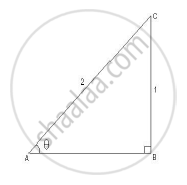SSC (Marathi Semi-English) 10thMaharashtra State Board
Share

# In the following figure, in ΔABC, BC = 1, AC = 2, ∠B = 90°. Find the value of sin θ. - SSC (Marathi Semi-English) 10th - Geometry

#### Question

In the following figure, in ΔABC, BC = 1, AC = 2, ∠B = 90°. Find the value of sin θ.#### Solution

In ΔABC, m∠B = 90°,

thereforesintheta=

therefore sintheta=1/2Is there an error in this question or solution?

#### APPEARS IN

2014-2015 (March) (with solutions)
Question 1.3 | 1.00 mark
Solution In the following figure, in ΔABC, BC = 1, AC = 2, ∠B = 90°. Find the value of sin θ. Concept: Heights and Distances.
S